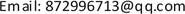1. 引言

2. 交通锥回收装置的系统组成与工作原理2.1. 机构组成

2.2. 工作流程

3. 总体设计3.1. 抓附机构设计

3.2. 传动机构设计

∵ μ m g cos θ > m g sin θ (1)

μ > tan θ (2)

∴ θ < arctan μ (3)

L = 5 ∗ d + 5 ∗ n = 3500 mm (4)

H = L sin θ = L sin 25.64 ∘ = 1515 mm (6)

3.3. 动力系统设计

∵ V 传 V 啮 = R 传 R 啮 = 0.5 ( 同 轴 ， ω 同 ) ∴ V 啮 = 2 km / h (7)

∵ V 车 V 动 = R 车 R 动 = 5 ( 同 轴 ， ω 同 ) ∴ V 啮 = 1 km / h (9)

V 动 ：工作车辆的行驶速度； V 动 ：与工作车辆同轴的动力轮的转动线速度； V 传 ：与动力轮外啮合的传动轮的转动线速度； V 啮 ：与传动轮同轴的齿轮的转动的线速度。

4. 结论